# Oops...

This website is made for modern browsers. You are seeing this, because your browser is missing a feature or two. Please install the latest update or switch to a modern browser. See you soon.

# Space frame of the Water Cube

In this tutorial, we will continue with the basics of Grasshopper and create another space frame, for which the National Aquatics Center will serve as inspiration. The center was built for the 2008 Olympic Summer Games in Bejing, China, and was nicknamed the Water Cube because of its iconic appearance.

The structural design of the Water Cube is derived from the structure of foam or, to be more precise, from an idealized representation of foam: the Weaire-Phelan structure. This model describes the idealized distribution of equal-sized bubbles within a soap film. The bubbles strive to minimize their surface area per volume and thus to have the smallest possible surface tension.

As you can see on the pictures below, the steel members of the space frame are arranged along the edges of the intersections between the bubbles. Between them, to cover the bubbles, there are ETFE cushions, which are also used to collect passive solar energy. The National Aquatics Center was design and built by a consortium of PTW Architects, Arup, CSCEC and CCDI.

See Wikipedia entry for Beijing National Aquatics Center.
See Wikipedia entry for Beijing National Aquatics Center.

The key element for the design of the space frame is creating of the foam structure. For this, we use a three-dimensional Voronoi tessellation, which follows the same principles as the Voronoi diagram

in a plane. We can imagine the generation of the voronoi as if balloons are inflated and as soon as they touch, they flatten each other. Thus, polyhedra are created inside the structure. For the generation of the Weaire-Phelan structure a weighted Voronoi (Laguerre Tessellation) should be used
Redenbach, Claudia & Sych, Tetyana. (2008). A RANDOM WEAIRE-PHELAN FOAM. Retrieved from researchgate.net.
Redenbach, Claudia & Sych, Tetyana. (2008). A RANDOM WEAIRE-PHELAN FOAM. Retrieved from researchgate.net., but for this tutorial a simple Voronoi tessellation should be sufficient.

## Grasshopper

To keep calculations easy, we will use smaller dimensions and larger elements for our replica of the Water Cube.

#### 1

##### Define a ground plan

The first thing we do in Grasshopper is to define the volume of our cube. To do this, we place the component Rectangle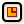Rectangle (Rectangle)
Curve  >  Primitive  >  Rectangle
Create a rectangle on a plane
Inputs
Plane (P)Rectangle base plane
X Size (X)Dimensions of rectangle in plane X direction.
Y Size (Y)Dimensions of rectangle in plane Y direction.
Outputs
Rectangle (R)Rectangle
Length (L)Length of rectangle curve
on the canvas to get a ground plan. We can assign the length and width of the rectangle at the inputs X and Y. But here, we need to provide domains. With these, we define from where to where, compared to the origin of the construction plane, the rectangle should spread out.

A domain can be created with the component Construct Domain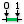Construct Domain (Dom)
Maths  >  Domain  >  Construct Domain
Create a numeric domain from two numeric extremes.
Inputs
Domain start (A)Start value of numeric domain
Domain end (B)End value of numeric domain
Outputs
Domain (I)Numeric domain between {A} and {B}
, where the inputs A and B represent the lower and upper limit. We create the numerical values with two PanelPanel
Params  >  Input  >  Panel
A panel for custom notes and text values
, one with 0 and the other with 30. A shortcut to create a domain is to write 0 To 30 directly into a PanelPanel
Params  >  Input  >  Panel
A panel for custom notes and text values
.

#### 2

##### Create the outer building volume

To create a volume with the just defined rectangle, we use Box Rectangle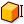Box Rectangle (BoxRec)
Surface  >  Primitive  >  Box Rectangle
Create a box defined by a rectangle and a height.
Inputs
Rectangle (R)Base rectangle
Height (H)Box height
Outputs
Box (B)Resulting box
and connect the rectangle from step 1 to the input R. The height at input H is again defined as a domain. If we connect a number instead of a domain (here 10), the component will automatically create a domain from 0 to the provided number; in this case the domain will be 0 To 10.

#### 3

##### Create the inner volume

To create a second, smaller volume, we use the component Offset Curve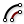Offset Curve (Offset)
Curve  >  Util  >  Offset Curve
Offset a curve with a specified distance.
Inputs
Curve (C)Curve to offset
Distance (D)Offset distance
Plane (P)Plane for offset operation
Corners (C)Corner type flag. Possible values:

none = 0
sharp = 1
round = 2
smooth = 3
chamfer = 4
Outputs
Curve (C)Resulting offsets
on the rectangle from before. The offset is set at input D and must be negative, in this example, so the offset will point inwards. The component Box RectangleBox Rectangle (BoxRec)
Surface  >  Primitive  >  Box Rectangle
Create a box defined by a rectangle and a height.
Inputs
Rectangle (R)Base rectangle
Height (H)Box height
Outputs
Box (B)Resulting box
will again turn the rectangle into a volume, on which we set the height a little smaller than before.

#### 4

##### Generate the Voronoi structure

Next, we want to generate a 3D Voronoi diagram between the two volumes. For this, we need the points around which the cells will be generated. We get them from creating random points in our larger volume with Populate 3DPopulate 3D (Pop3D)
Vector  >  Grid  >  Populate 3D
Populate a 3-Dimensional region with points
Inputs
Region (R)Box that defines the 3D region for point insertion
Count (N)Number of points to add
Seed (S)Random seed for insertion
Points (P)Optional pre-existing population
Outputs
Population (P)Population of inserted points
. On this component, the larger volume goes into input R, which defines the region that will contain the random points. At input N we define the number of points. Bear in mind that a large number increases the calculation time later on.

Now, the just created points will serve us as input P (Points) on a Voronoi 3D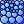Voronoi 3D (Voronoi³)
Mesh  >  Triangulation  >  Voronoi 3D
Volumetric voronoi diagram for a collection of points
Inputs
Points (P)Points for Voronoi diagram
Box (B)Optional diagram boundary
Outputs
Cells (C)Cells of the 3D Voronoi diagram
Boundary (B)List of boolean values indicating for each cell whether it is part of the original boundary
component. At input B we connect the larger volume, so that the Voronoi cells are created within this Box.

#### 5

##### Cut the Voronoi cells

After having a look at the cells in Rhino, we notice that they fill the whole volume, even the inner, smaller one, which is not what we desire. Therefore, we have to cut the inner volume out of the Voronoi foam. For this we use Solid Difference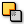Solid Difference (SDiff)
Intersect  >  Shape  >  Solid Difference
Perform a solid difference on two Brep sets.
Inputs
Breps A (A)First Brep set
Breps B (B)Second Brep set
Outputs
Result (R)Difference result
: Connect the cells with input A and the smaller volume with input B. Now, the components calculates the difference and we get two lists with Breps.

#### 6

##### Select the desired results

The cutting process worked for all cells that touched the inner volume, but we still have some isolated, intact cells inside the smaller volume. Let’s filter them out: For this we determine the center point of each Brep with VolumeVolume (Volume)
Surface  >  Analysis  >  Volume
Solve volume properties for closed breps and meshes.
Inputs
Geometry (G)Closed brep or mesh for volume computation
Outputs
Volume (V)Volume of geometry
Centroid (C)Volume centroid of geometry
and check with Point In Brep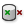Point In Brep (BrepInc)
Surface  >  Analysis  >  Point In Brep
Test whether a point is inside a closed brep
Inputs
Brep (B)Brep for inclusion test
Point (P)Point for inclusion test
Strict (S)If true, then the inclusion is strict
Outputs
Inside (I)True if point is on the inside of the Brep.
whether the center points is within the boundary of the smaller volume. Thus, we connect the smaller volume with input B and the points with input P. At output we get a list with Boolean variables.

Now, we use Dispatch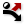Dispatch (Dispatch)
Sets  >  List  >  Dispatch
Dispatch the items in a list into two target lists.
Inputs
List (L)List to filter
Dispatch pattern (P)Dispatch pattern
Outputs
List A (A)Dispatch target for True values
List B (B)Dispatch target for False values
to filter all inner Voronoi cells: We connect the output from Solid DifferenceSolid Difference (SDiff)
Intersect  >  Shape  >  Solid Difference
Perform a solid difference on two Brep sets.
Inputs
Breps A (A)First Brep set
Breps B (B)Second Brep set
Outputs
Result (R)Difference result
with input L and our list of Boolean values with input P (Pattern).
Have a look at Filter lists for more on working with lists.
Have a look at Filter lists for more on working with lists. We receive a list of cells that lie between the two volumes at output B. For these, the check, if they are inside the smaller volume, returned False.

#### 7

##### Extract the structural elements

In the final step, we can bundle the Voronoi cells inside a BrepBrep (Brep)
Params  >  Geometry  >  Brep
Contains a collection of Breps (Boundary REPresentations)
container to get the cell walls. We can also connect our result to a Brep Wireframe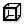Brep Wireframe (Wires)
Surface  >  Analysis  >  Brep Wireframe
Extract the wireframe curves of a brep.
Inputs
Brep (B)Base Brep
Density (D)Wireframe isocurve density
Outputs
Wireframe (W)Wireframe curves
component to extract the edges from the cells. These edges can now serve as axes for our supporting member of the space frame.

## Get the results

### Version Info

• Rhino 6.30
• Grasshopper 1.0.0007